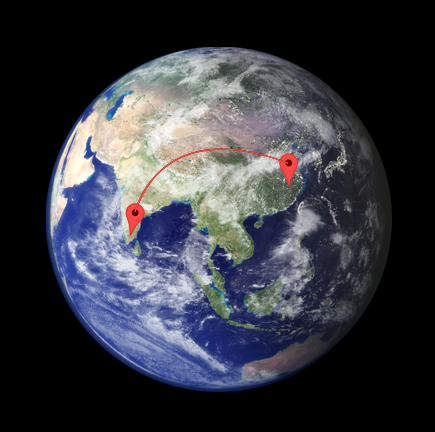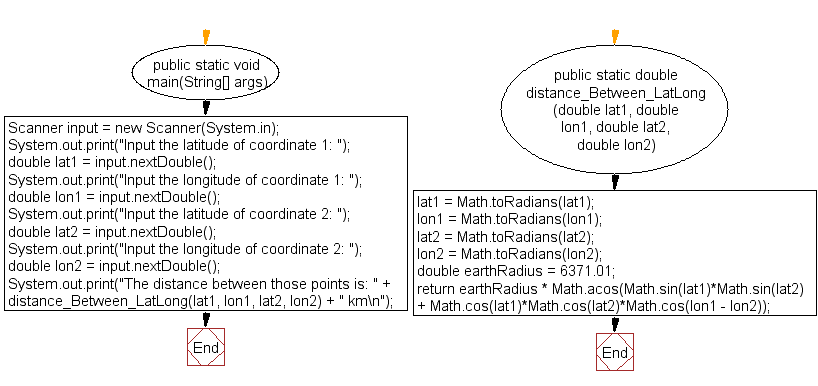﻿ Java: Distance between two points on the surface of earth# Java Exercises: Compute the distance between two points on the surface of earth

## Java Basic: Exercise-36 with Solution

Write a Java program to compute the distance between two points on the surface of earth.

Distance between the two points [ (x1,y1) & (x2,y2)]
d = radius * arccos(sin(x1) * sin(x2) + cos(x1) * cos(x2) * cos(y1 - y2))
Radius of the earth r = 6371.01 Kilometers

Test Data:
Input the latitude of coordinate 1: 25
Input the longitude of coordinate 1: 35
Input the latitude of coordinate 2: 52.5
Input the longitude of coordinate 2: 35.5

Pictorial Presentation:Sample Solution:

Java Code:

``````import java.util.Scanner;

public class Exercise36 {

public static void main(String[] args) {

Scanner input = new Scanner(System.in);
System.out.print("Input the latitude of coordinate 1: ");
double lat1 = input.nextDouble();
System.out.print("Input the longitude of coordinate 1: ");
double lon1 = input.nextDouble();
System.out.print("Input the latitude of coordinate 2: ");
double lat2 = input.nextDouble();
System.out.print("Input the longitude of coordinate 2: ");
double lon2 = input.nextDouble();

System.out.print("The distance between those points is: " + distance_Between_LatLong(lat1, lon1, lat2, lon2) + " km\n");
}

// Points will be converted to radians before calculation
public static double distance_Between_LatLong(double lat1, double lon1, double lat2, double lon2) {

return earthRadius * Math.acos(Math.sin(lat1)*Math.sin(lat2) + Math.cos(lat1)*Math.cos(lat2)*Math.cos(lon1 - lon2));
}
}
```
```

Sample Output:

```Input the latitude of coordinate 1: 25
Input the longitude of coordinate 1: 35
Input the latitude of coordinate 2: 52.5
Input the longitude of coordinate 2: 35.5
The distance between those points is: 3058.15512920181 km
```

Flowchart:Java Code Editor:

What is the difficulty level of this exercise?

Test your Programming skills with w3resource's quiz.

﻿

## Java: Tips of the Day

countOccurrences

Counts the occurrences of a value in an array.

Use Arrays.stream().filter().count() to count total number of values that equals the specified value.

```public static long countOccurrences(int[] numbers, int value) {
return Arrays.stream(numbers)
.filter(number -> number == value)
.count();
}
```

Ref: https://bit.ly/3kCAgLb